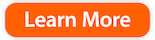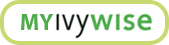Tips and Free Tools For Students Using Calculators On the SAT

By Priyam, IvyWise Master Tutor

It’s SAT and ACT season for college bound students, and many will go into these tests with a calculator in-hand. Make sure you know how – and when – to use it.

While the College Board's official calculator policy states that you do not need a calculator for the SAT, the reality is that a calculator is an absolute must for the exam. Specifically, calculators are allowed on the Math Section 4 of the SAT (not on the Math Section 3) and not using them correctly can be a disadvantage over other savvy test takers.

However, you need to have the right SAT calculator strategy while interpreting the problems and you can't expect the calculator to perform magic for you during the exam.

What Is the Best Calculator For Me?

At IvyWise, we recommend investing in a TI-83 (discontinued) or TI-84, which are the most popular graphing calculator used for standardized exams. It offers advanced calculation, graphing, statistics, and scientific functions that will be more than sufficient for the SAT.Other more advanced calculators (i.e. TI-89) offer symbolic math functions, calculus tools, and the ability to graph in 3D. The SAT does not specify which graphing calculators to use or whether calculator programs are allowed so the current interpretation is that you can use programmable calculators. However, rules can change so double check the rules before you go into the exam.

In order to get the most out of your calculator, you must be familiar with it and have used ideally for 20 hours or more. This helps ensure that you have a vague muscle memory of where the keys are, what settings there are, and so forth. Hopefully you use the calculator you already use for school work and homework.

Calculator Features

Doing basic calculations (adding, subtraction, multiplying, dividing, exponent, radical, logarithm) that are otherwise time consuming is an invaluable use of your calculator. If you are unfamiliar with where these crucial keys are on the calculator, take time to understand where they are. Additionally, there are many other in-built functions and features on your graphing calculator that are also incredibly helpful.

MATH Button

Hitting the Math button (as seen below) unveils the Frac and Dec functions, which turns decimals to fractions or fractions to decimals.For example, let us say you are doing the following calculation which yields: 1/2 + 2/5 + 4/7 = 1.4714285. However, if the answer choices are all in fractions, this could mean additional work. The Frac function helps convert decimal to fraction form and the Dec function converts fractions to decimals.

Graphing
System of complex equations and quadratics can be easily graphed on calculators as well as absolute value, inequalities, parabolas, ellipses, trig functions and a whole host of other more complex equations that are difficult to draw by hand and do the required calculation.

There are many downloadable programs on www.ticalc.org that can quickly allow factoring, foiling, use information to create slope-intercept or point slope form equations, determine values of unknown variables in systems of equations, and even roots of quadratics. Such quick calculation short-cuts can save time and makes your life easier by adding accuracy.

In order to access these programs, simply visit  www.ticalc.org and pick and choose which programs to download into your calculator. Pick the graphing calculator you have from the list, connect the link cable which the calculator came with to your computer and simply download the programs you think you will need. There are hundreds of programs, games and applications to choose from but for the purposes of the SAT – pick the programs that you will be able to use.

At the end of the day, keep in mind that the calculator is there to help you when necessary. Your test prep plan should include test-taking strategies that help you identify when you need a calculator and when you don’t. Do not attempt to use a calculator for a problem that could easily be done in your head or on paper because it will eat into the time you need to think through and analyze the problem.

Calculators can be very helpful tools when taking the SAT or ACT, but know when they’re needed and when they’re not!

For more test prep tips from our team of expert tutors, download our free Tutoring and Test Prep Checklist below!